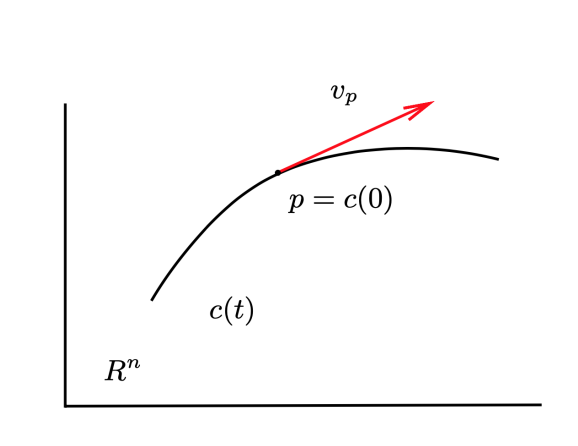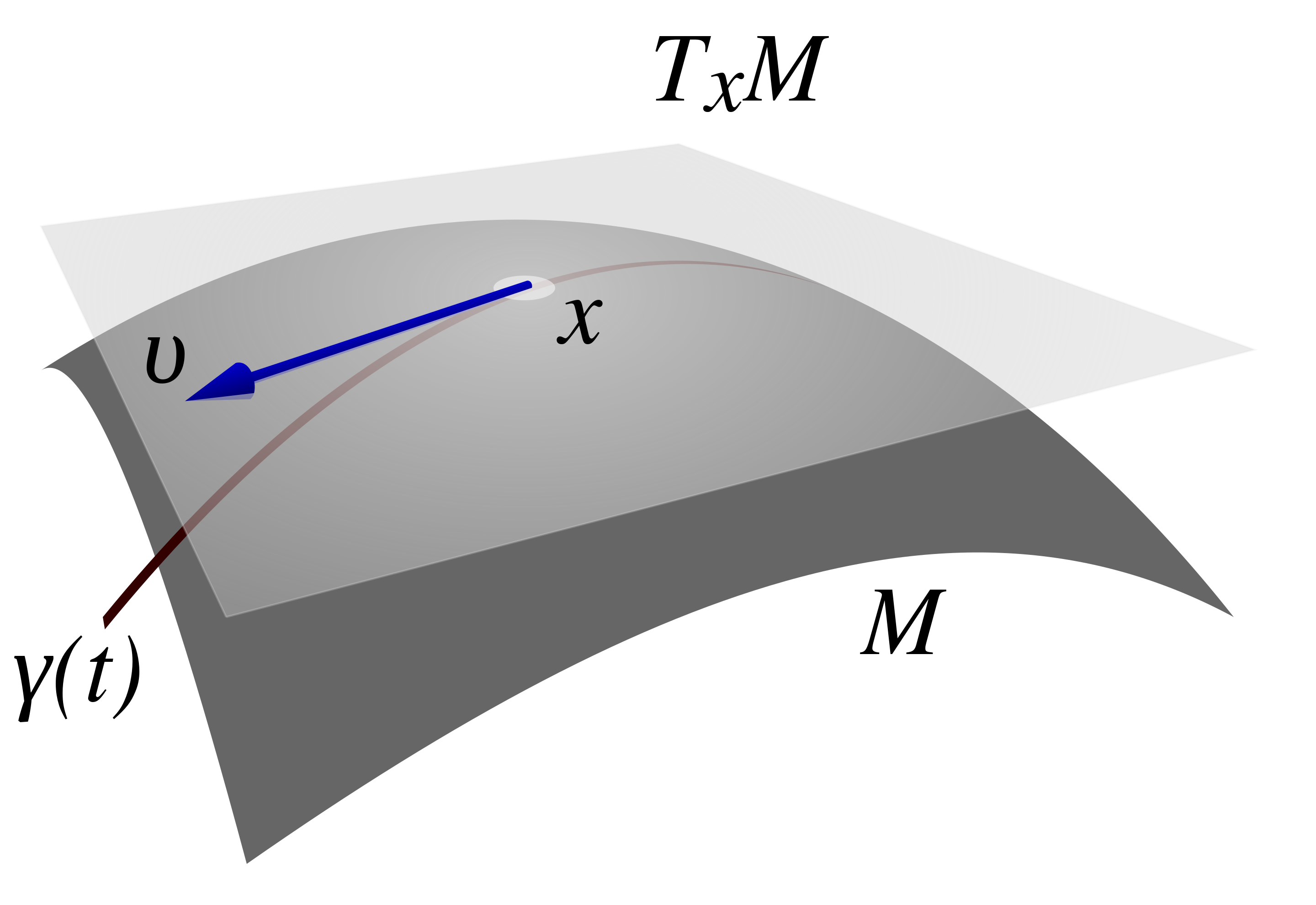$\varphi: U\rightarrow \mathbb{R}^n$

$(x^1 (p),\cdots ,x^ n (p))$

\begin{aligned} &x^i : \mathbb{R}^n \rightarrow \mathbb{R}\\ &u \mapsto x^i (u) = (u)^i ,\ \forall u \in \mathbb{R}^n \end{aligned}

\begin{aligned} (\varphi(p))^i = x^i(\varphi(p)) = x^i\circ\varphi(p) = x^i_{\varphi}(p)\\ \end{aligned}

$c: I\subset\mathbb{R} \rightarrow X$

$X$ 的坐标卡 $(U,\varphi)$ 下，该曲线可表示为：

$x ^ i = (\varphi \ \circ\ c(t))^ i ,\quad i = 1,\cdots ,n$

$x^i = c^i(t) \ or\ x^i = x^i(t)$

• 一般的微分流形不具备线性空间这样的代数结构。因此，给定一个一般的微分流形后，我们不可能像欧氏空间那样定义一个矢量。
• 欧几里德空间中曲线和曲面的切矢量，可以通过将一个微分流形嵌入高维的欧氏空间来定义切矢量。但这种定义依赖于微分流形的非内禀结构（需要将流形嵌入到一个高维的流形，如嵌入到高维的欧几里德空间）。例如一个对于一个二维流形，我们总可以将其嵌入到三维欧氏空间中，并定义相应的切矢量：## 切空间

\begin{aligned} &v_p(\lambda_1f_1 + \lambda_2f_2) = \lambda_1v_p(f_1) + \lambda_2v_p(f_2)\\ &\forall\ \lambda_1,\lambda_2\in \mathbb{R},\quad \forall\ f_1,f_2\in\mathscr{F}(X) \end{aligned}

$v_p (f_ 1 f_ 2 ) = v_ p (f_ 1 )f_ 2 + f_ 1 v_ p (f_ 2 )$$c : (-\epsilon,\epsilon) \rightarrow X$ 为流形 $X$ 上过 $p$ 点的一条曲线，其中 $c(0) = p$。则这条曲线给出一个映射：

$v_p:\mathscr{F}(X) \rightarrow \mathbb{R}$

$v_p(f)=\frac{d(f\circ c(t))}{dt}|_ {t=0},\ \forall f\in \mathscr{F}(X)$

$f\circ c:(-\epsilon,\epsilon) \rightarrow \mathbb{R}$

$c_j: (-\epsilon,\epsilon)\rightarrow X, j=1,\cdots,n$

$x^i(c_j(t)) = x^i(p_0) + t\delta^i_j$

\begin{aligned} e_j(f) &= \frac{d(f\circ c_j(t))}{dt}|_{t=0}\\ &= \frac{d}{dt}(f\circ \varphi^{-1}\circ \varphi \circ c_j(t))|_{t=0}\\ &= \frac{d}{dt}(f\circ \varphi^{-1}(\varphi \circ c_j(t)))|_{t=0}\\ &= \frac{\partial(f\circ \varphi^{-1})}{\partial x^k}(\varphi\cdot c_j(t))\cdot \frac{dx^k(c_j(t))}{dt}|_{t=0}\\ &= \frac{\partial(f\circ \varphi^{-1})}{\partial x^k}|_{\varphi(p_0)}\delta^k_j\\ &= \frac{\partial(f\circ \varphi^{-1})}{\partial x^j}|_{\varphi(p_0)} \end{aligned}

$(\varphi\circ c_j(t))^k = x^k(c_j(t))$

$e_j(f)=\frac{\partial (f\circ \varphi^{-1})}{\partial x^j}|_ {\varphi(p_0)}$

$e_i$ 在函数 $f$ 上的作用等价于其表示函数 $F = f\circ \varphi^{-1}$$\varphi(p_0 )$$x_i$ 的偏导数。

$e_j (x^i) = \delta^i_{j}$

$x_0\in \mathbb{R}^n, F\in \mathscr{F}(\mathbb{R}^n)$，则 $F$ 可以表示为：

$F(x) = F(x_0) + (x^i-x_0^i)\frac{\partial F}{\partial x^i}|_{x_0}$

$F = f\circ \varphi^{-1}:\ V\rightarrow \mathbb{R}$

\begin{aligned} f(p) &= F(\varphi(p)) = F(\varphi(p_0)) + (x^i(p)-x^i(p_0))\frac{\partial F}{\partial x^i}|_{f(p_0)}\\ &= f(p_0) + (x^i(p)-x^i(p_0))\frac{\partial F}{\partial x^i}|_{f(p_0)}\\ \end{aligned}

$v_{p_0}$ 作用到 $f$ 的这一表达式上得到：

$v_{p_0}(f) = v_{p_0}(x^i)\frac{\partial F}{\partial x^i}|_{\varphi(p_0)} = v_{p_0}(x^i)\frac{\partial (f\circ \varphi^{-1})}{\partial x^i}|_{\varphi(p_0)}$

$v_{p_0}(f) = v_{p_0}(x^i)e_i(f)$

$v_{p_0} = v_{p_0}(x^i)e_i = v_{p_0}^ie_i$

$a^ie_i(x^j) = a^i\delta^{j}_{i} = a^j = 0$

$\{ e_i=\frac{\partial}{\partial x^i}|_ p,i = 1,\cdots ,n \}$

$v_p^i$ 是切矢量 $v_p$ 在这个自然基下的分量。

$v_p=\frac{dx^i(c(t))}{dt}| _ {t=0} \frac{\partial}{\partial x^i}|_ p$

$(\tilde{U},\tilde{\varphi})$ 是另外一个包含点 $p$ 的坐标卡，而相应的坐标函数为 $\tilde{x}^i$，因此 $p$ 点的任意一个切矢量 $v_p$ 可以展开为：

$v_p = v_p^ie_i = \tilde{v}_p^i\tilde{e}_i = \tilde{v}_p^i\frac{\partial}{\partial \tilde{x}^i}|_p$

$\tilde{e}_i = \tilde{e}_i(x^j)e_j = \frac{\partial (x^j \circ \tilde{\varphi}^{-1})}{\partial\tilde{x}^i}|_{\tilde{\varphi}(p)}e_j$

$v_p^j = A^j_{\ \ i}\tilde{v}^i_p$

$A^j_{\ \ i} = \frac{\partial (x^j \circ \tilde{\varphi}^{-1})}{\partial\tilde{x}^i}|_{\tilde{\varphi}(p)} = \frac{\partial (\varphi \circ \tilde{\varphi}^{-1})^j}{\partial\tilde{x}^i}|_{\tilde{\varphi}(p)}$

\begin{aligned} x^j(p) &= (\varphi\circ \tilde{\varphi}^{-1})^j(\tilde{\varphi}(p))\\ &= (\varphi\circ \tilde{\varphi}^{-1})^j(\tilde x^1(p),\cdots,\tilde{x}^n(p))\\ \end{aligned}

$x^j$$(\tilde{x}^1,\cdots,\tilde{x}^n)$ 的函数，我们可以将 $A^{j}_{\ \ i}$ 简记为：

$A^j_{\ \ i} = \frac{\partial x^j}{\partial \tilde{x}^i} = \frac{\partial x^j(\tilde{x}^1,\cdots,\tilde{x}^n)}{\partial \tilde{x}^i}$

$v_p^j = A^j_{\ \ i}\tilde{v}^i_p$

## 余切空间

$v_p:f\mapsto v_p(f) \in \mathbb{R}$

$df|_ p : v_p \mapsto df| _ p (v_p ) = v_p (f)$

$(U,\varphi)$ 是包含 $p$ 的坐标卡，且坐标函数为 $x^i$，有 $x^i\in \mathscr{F}(X)$，从而我们得到如下 $n$ 个余切矢量：

$dx^i|_p(v_p) = v_p(x^i)$

$dx^i|_p(\frac{\partial}{\partial x^j}|_p) = \frac{\partial}{\partial x^j}|_p (x^i) = \delta^i_j$

$\{dx^i|_p,i=1,\cdots,n\}$

$\{\frac{\partial}{\partial x^i}|_p,i=1,\cdots,n\}$ 的对偶基。我们将其记为：

$\{e^{* i}=dx^i | _ p ,i = 1,\cdots ,n\}$

$\omega_p = \omega_p(e_i) e^{*i} = \omega_{pi}e^{*i}$

$df|_p = df|_p(e_i)e^{*i} = df|_p(\frac{\partial}{\partial x^i}|_p)e^{*i} = \frac{\partial f}{\partial x^i}| _ p dx^i| _ p$

$\frac{\partial \tilde{x}^j}{\partial x^i}\tilde{\omega}_{pj} = (A^{-1})^j_{\ \ i}\tilde{\omega}_{pj} = \omega_{pi}$